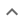•# Adding, subtracting and finding the least common multiple

We think you wrote:

###This solution deals with adding, subtracting and finding the least common multiple.

Solution found(-4)/((m+7)*(m+3))

## Step by Step Solution## Step  1  :

              1
Simplify   —————
m + 3


#### Equation at the end of step  1  :

     1         1
——————— -  —————
(m + 7)    m + 3


## Step  2  :

              1
Simplify   —————
m + 7


#### Equation at the end of step  2  :

    1        1
————— -  —————
m + 7    m + 3


## Step  3  :

#### Calculating the Least Common Multiple :

Find the Least Common Multiple

The left denominator is :       m+7

The right denominator is :       m+3

Number of times each Algebraic Factor
appears in the factorization of:
Algebraic
Factor
Left
Denominator
Right
Denominator
L.C.M = Max
{Left,Right}
m+7 101
m+3 011

Least Common Multiple:
(m+7) • (m+3)

#### Calculating Multipliers :

Calculate multipliers for the two fractions

Denote the Least Common Multiple by  L.C.M
Denote the Left Multiplier by  Left_M
Denote the Right Multiplier by  Right_M
Denote the Left Deniminator by  L_Deno
Denote the Right Multiplier by  R_Deno

Left_M = L.C.M / L_Deno = m+3

Right_M = L.C.M / R_Deno = m+7

#### Making Equivalent Fractions :

Rewrite the two fractions into equivalent fractions

Two fractions are called equivalent if they have the same numeric value.

For example :  1/2   and  2/4  are equivalent,  y/(y+1)2   and  (y2+y)/(y+1)3  are equivalent as well.

To calculate equivalent fraction , multiply the Numerator of each fraction, by its respective Multiplier.

   L. Mult. • L. Num.           m+3
——————————————————  =   —————————————
L.C.M             (m+7) • (m+3)

R. Mult. • R. Num.           m+7
——————————————————  =   —————————————
L.C.M             (m+7) • (m+3)


#### Adding fractions that have a common denominator :

Adding up the two equivalent fractions
Add the two equivalent fractions which now have a common denominator

Combine the numerators together, put the sum or difference over the common denominator then reduce to lowest terms if possible:

  m+3 - (m+7)              -4
—————————————  =  —————————————————
(m+7) • (m+3)     (m + 7) • (m + 3)


## Final result :

          -4
—————————————————
(m + 7) • (m + 3)